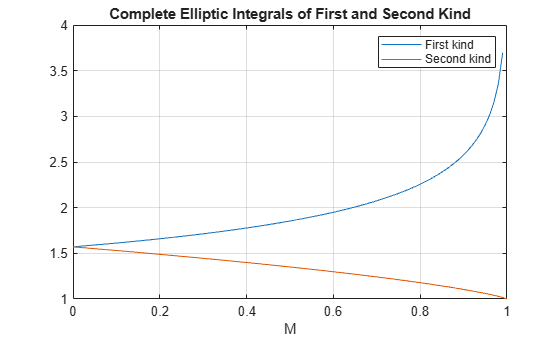# ellipke

Complete elliptic integrals of first and second kind

## Syntax

``K = ellipke(M)``
``````[K,E] = ellipke(M)``````
``````[K,E] = ellipke(M,tol)``````

## Description

````K = ellipke(M)` returns the complete elliptic integral of the first kind for each element in `M`.```

example

``````[K,E] = ellipke(M)``` returns the complete elliptic integral of the first and second kind.```

example

``````[K,E] = ellipke(M,tol)``` computes the complete elliptic integral to accuracy `tol`. The default value of `tol` is `eps`. Increase `tol` for a less accurate but more quickly computed answer.```

## Examples

collapse all

Find the complete elliptic integrals of the first and second kind for `M = 0.5`.

```M = 0.5; [K,E] = ellipke(M)```
```K = 1.8541 ```
```E = 1.3506 ```

Plot the complete elliptic integrals of the first and second kind for the allowed range of `M`.

```M = 0:0.01:1; [K,E] = ellipke(M); plot(M,K,M,E) grid on xlabel('M') title('Complete Elliptic Integrals of First and Second Kind') legend('First kind','Second kind')```The default value of `tol` is `eps`. Find the runtime with the default value for arbitrary `M` using `tic` and `toc`. Increase `tol` by a factor of thousand and find the runtime. Compare the runtimes.

```tic ellipke(0.904561)```
```ans = 2.6001 ```
`toc`
```Elapsed time is 0.029776 seconds. ```
```tic ellipke(0.904561,eps*1000)```
```ans = 2.6001 ```
`toc`
```Elapsed time is 0.011964 seconds. ```

`ellipke` runs significantly faster when tolerance is significantly increased.

## Input Arguments

collapse all

Input array, specified as a scalar, vector, matrix, or multidimensional array. `M` is limited to values 0≤m≤1.

Data Types: `single` | `double`

Accuracy of result, specified as a nonnegative real number. The default value is `eps`.

Data Types: `single` | `double` | `int8` | `int16` | `int32` | `int64` | `uint8` | `uint16` | `uint32` | `uint64`

## Output Arguments

collapse all

Complete elliptic integral of the first kind, returned as a scalar, vector, matrix, or multidimensional array.

Complete elliptic integral of the second kind, returned as a scalar, vector, matrix, or multidimensional array.

collapse all

### Complete Elliptic Integrals of the First and Second Kind

The complete elliptic integral of the first kind is

`$\left[K\left(m\right)\right]={\int }_{0}^{1}{\left[\left(1-{t}^{2}\right)\left(1-m{t}^{2}\right)\right]}^{-\frac{1}{2}}dt.$`

where m is the first argument of `ellipke`.

The complete elliptic integral of the second kind is

`$E\left(m\right)={\int }_{0}^{1}\left(1-{t}^{2}{\right)}^{-\frac{1}{2}}{\left(1-m{t}^{2}\right)}^{\frac{1}{2}}dt.$`

Some definitions of the elliptic functions use the elliptical modulus k or modular angle α instead of the parameter m. They are related by

`${k}^{2}=m={\mathrm{sin}}^{2}\alpha .$`

 Abramowitz, M., and I. A. Stegun. Handbook of Mathematical Functions. Dover Publications, 1965.